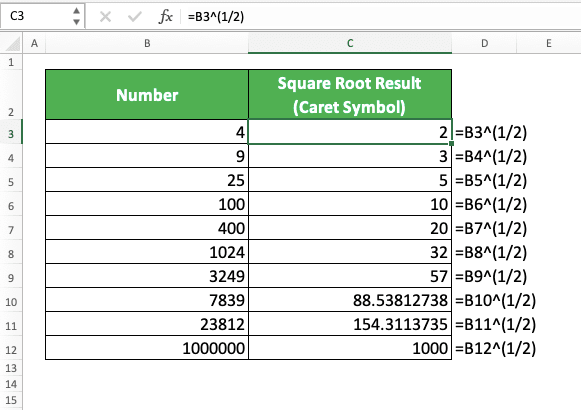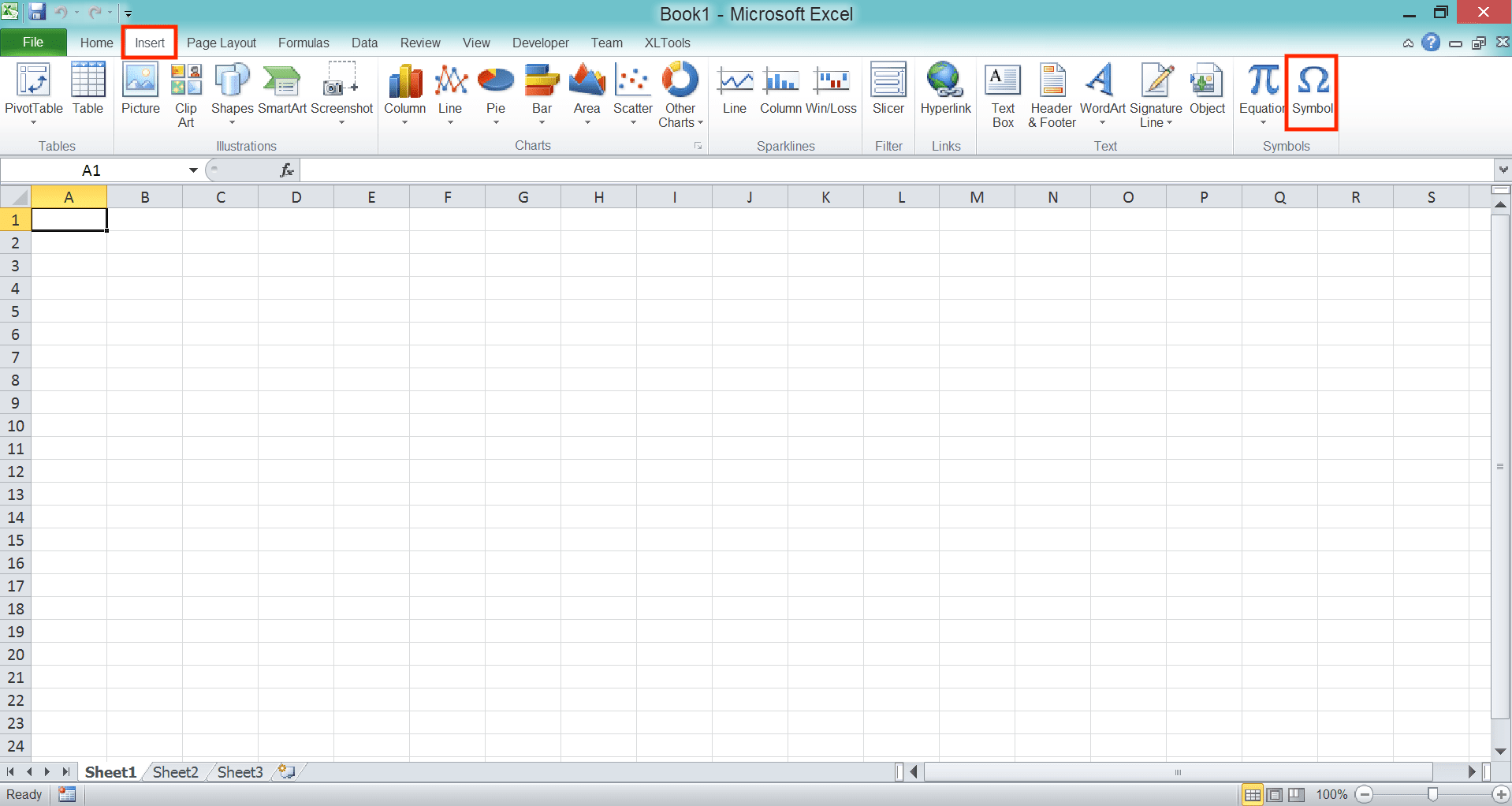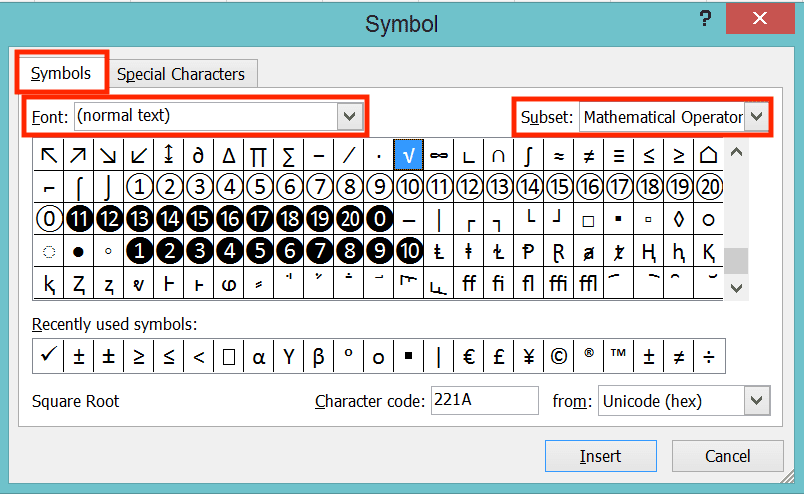How to Calculate Square Root in Excel - Compute Expert

# How to Calculate Square Root in Excel

Home >> Excel Tutorials from Compute Expert >> Excel Calculations >> How to Calculate Square Root in Excel

In this tutorial, you will learn how to calculate square root in excel completely.

When processing number data in excel, a square root calculation is one of the things we sometimes need to do. There are several methods to do this kind of calculation in excel. You should master at least one of them so you can do calculation on your numbers optimally.

Want to know what those square root calculation methods are and how to implement them in excel? Learn from this tutorial until its last part!

Disclaimer: This post may contain affiliate links from which we earn commission from qualifying purchases/actions at no additional cost for you. Learn more

## What is a Square Root?

A square root is the second level of root calculation. It is the opposite of the square calculation.

The standard writing of a square root calculation is like this.The x there is the number we want to square root.

If we square a number, then that means we multiply a number by itself to find the square result. If we square root a number, then we try to find what number we multiply by itself that produces that number.

For example, let’s say we want to square root the number of 9. That means we try to find what number we multiply by itself will produce 9. The answer for that is 3 (because 3 multiplies by 3 equals 9).

It can be hard to calculate the square root of a number manually. However, in excel, we can use several methods to do the calculation automatically!

There are three square root calculation methods we will discuss in this tutorial. The first one is probably the simplest one, which is by using the SQRT formula.

## Method 1: Calculate Square Root In Excel by Using the SQRT Formula

SQRT is a formula excel provides specifically to square root a number. Just input the number you want to square root in it and you are good to go.

Because it only needs your number as its input, the general writing form of SQRT becomes like this.

= SQRT ( number_to_square_root )

Simple, isn’t it? And here is the implementation example of the formula in excel.As you can see, we just need to input the number we want to square root into SQRT. Do that and we will immediately get our square root result!

## Method 2: Calculate Square Root In Excel by Using the POWER Formula

Another way to calculate square root in excel is by using the POWER formula. However, this method is a bit more complicated if we compare it to the SQRT method.

POWER is a formula excel provides to power a number in its software. However, since root is the opposite of power, then we can use it to calculate square root too. Just input the power level of half in POWER (because the square root is the opposite of square, we use the opposite power level of square (2) which is half (1/2)) and you are good to go.

Based on that, here is the general writing form of POWER in excel to calculate square root.

= POWER ( number_to_square_root , 1/2 )

Just input the number we want to square root and a half number to POWER. You can input the decimal form of half (0.5) for the power level input too if you want.

You can see the POWER implementation example to calculate square root in excel below. We use the same set of numbers that we use in the SQRT formula implementation example earlier.As you can see, we get the same results as the SQRT method too by using POWER. That is because we use half as the input of our POWER. That makes the formula square root a number instead of powering it.

## Method 3: Calculate Square Root In Excel by Using the Caret Symbol (^)

This last method also uses the principle that the root is the opposite of power in its calculation process. As we can use caret (^) to power a number, that means we can calculate square root by using it too.

As with the POWER formula previously, we also use half as the power level input in our writing. Here is the general writing form of square root calculation in excel using the caret symbol.

= number_to_square_root ^ ( 1 / 2 )

We use a bracket there so the power calculation won’t run before we divide one with two for our power level. If you don’t want to have brackets, then you can input 0.5 instead as the power level.

Here is the implementation example of this method in excel.Just write an equal symbol, the number you want to square root, a caret symbol, and a half. You will immediately get your square root result!

## How to Calculate Nth Root in Excel

If you want to have another root level calculation, then you can use POWER or the caret symbol. Just divide one with the root level you want for your calculation as the power level input when you write them.

In general, here is the writing form of the POWER and caret symbol methods to calculate nth root in excel.

POWER

= POWER ( number_to_root , n )

Caret Symbol

= number_to_root ^ ( 1 / n )

Similar to the square root calculation writing form, isn’t it? The n there is the root level for your calculation.

To better understand the concept, here is the implementation example in excel.## How to Insert a Square Root Symbol in Excel

Need to insert a square root symbol instead of doing its calculation?

There are several methods to insert a square root symbol in excel. We will discuss the three of them here: the Insert Symbol menu, the UNICHAR formula, and the Alt button.

The first one is by using the Insert Symbol menu. Unfortunately, this only seems to work for you who use Windows OS as Mac seems to not have the symbol.

If you are a Windows user, then go to the Insert tab and click the Symbol button there.In the dialog box that shows up, make sure you are in the Symbols tab. On the top of the dialog box, choose (normal text) from the Font dropdown and Mathematical Operators from the Subset dropdown.Then, on the bottom, choose Unicode (hex) from the from dropdown and type 221A in the Character code text box. This will make you highlight the square root symbol from the symbol choices in the dialog box.Double-click the square root symbol or click the Insert button in the dialog box. Excel will immediately insert a square root symbol to your current active cell!

Another method you can use is by using the UNICHAR formula. This formula is available to use since excel 2013.

If you have excel 2013 onwards, then you can just type this in your cell to get a square root symbol.

= UNICHAR ( 8730 )

The 8730 there is the code input for UNICHAR to produce a square root symbol for us. You can use the ampersand symbol (&), CONCATENATE, CONCAT, or TEXTJOIN if you want to add to the symbol writing (e.g. to type “9=3” after the square root symbol, you can write like this: =UNICHAR(8730)&9&”=“&3)).

The last method is by using the Alt button. You can implement this method if you have a keyboard with a numeric keypad.

A numeric keypad, by the way, is a keypad with numbers. It is usually on the right side of your keyboard (not the usual numbers keypad on the near top of your keyboard).

If you have that, then make sure your NumLock is on to implement this method. After that, just hold Alt while pressing 2, 5, and 1 in the numeric keypad. You will immediately insert a square root symbol into your current active cell!

So, which one of the square symbol insertion methods that you prefer to use?

## Exercise

After you have learned the methods to calculate square root in excel, now let’s do an exercise. You should do this so you can deepen your understanding of those methods.

### Questions

Answer in the appropriate gray-colored cells!
1. What is the result from the three square root calculation methods implementation on the first number?
2. What is the result from the three square root calculation methods implementation on the second number?
3. What is the result from the three square root calculation methods implementation on the third number? Round those results to two decimal places! (Learn how to round numbers in excel by visiting this tutorial!)

You cannot square root a negative number as there isn’t a number multiplication with itself that produces that. You will get a #NUM error if you try to square root a negative number in excel.

Related tutorials for you to learn:

Get updated excel info from Compute Expert by registering your email. It's free!